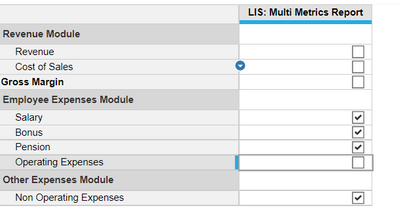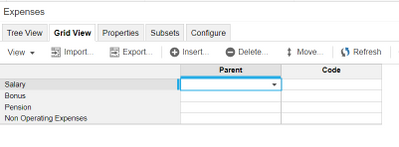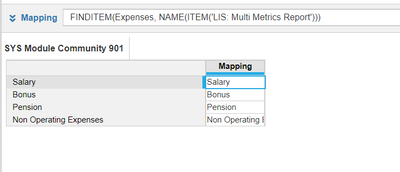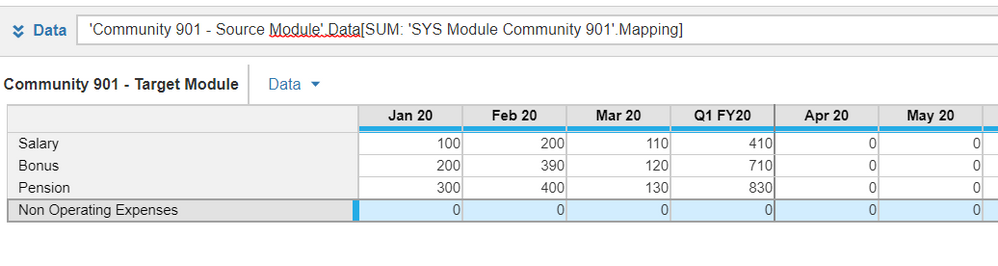# Summary over Line Item SubsetHello all,

I need to calculate a sum of costs from P&L which are base for subsequent calculation of revenue (sum of costs * (1+margin%)). The tricky part is that we need dynamic setting for identifying the costs to be aggregated because each year different cost might be considered as part of the base. I would therefore like to avoid extensive use of IF function and hardcoding.
Instead, I would like to do that using Line item subset (LIS) which is easy to configure. I wanted to go about this in the following way which unfortunately does not work because LIS does not have a top level.

Step 1: Collect the costs from P&L module [dimensions: Departments, Geography, LIS, Months]

Step 2: Sum up the costs to calculate the Base [dimensions: Departments, Geography, Months]

Do you know of possible workaround?
Tagging @JaredDolich @Misbah @rob_marshall in case if you have any suggestions.

Tagged:

•See if this helps

Step 1: Create a LIS - I assume you have already createdStep 2: Create a List mimicking LISStep 3: Create a SYS module for the mapping between LIS from Step 1 and List from Step 2Step 4: Use SUM function to aggregate the values from source module. Target module will have "Expenses List" as a dimensionThis process basically allows you to aggregate the values out of LIS because there is no TOP Level for LIS

Misbah